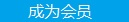您可以捐助，支持我们的公益事业。 1元 10元 50元 认证码：必填求知 文章 文库 Lib 视频 iProcess 课程 认证 咨询 工具 讲座 Modeler Code要资料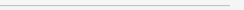订阅捐助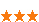1377 次浏览     评价： 好 中 差
2018-9-29

 编辑推荐: 本文来自于csdn，本文将通过以OCR（光学字符识别）的场景来介绍深度学习在计算机视觉中的应用。

1. 数据分布，x轴是属性城市人口，y轴是标签值盈利：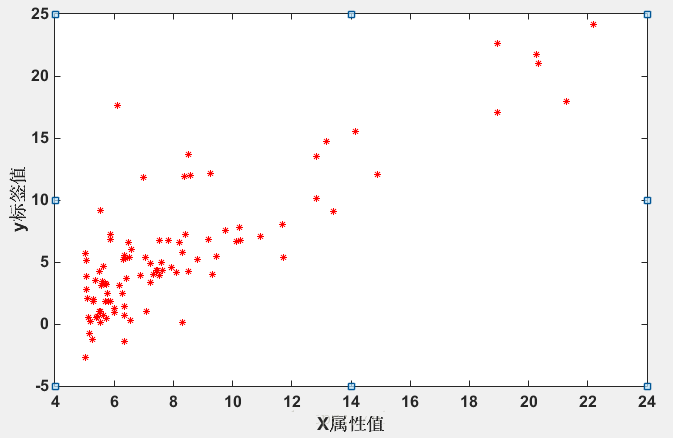2. 目的：使用一个线性函数去拟合上面这些数据；

1）设置线性函数，即假设函数(Hypothesis)；

2）选定损失函数 J，

3）梯度下降，找到使得J值最小时，对应的theta_0, theta_1。

J值最小，预测的h_theta(x)值就会越接近标签纸y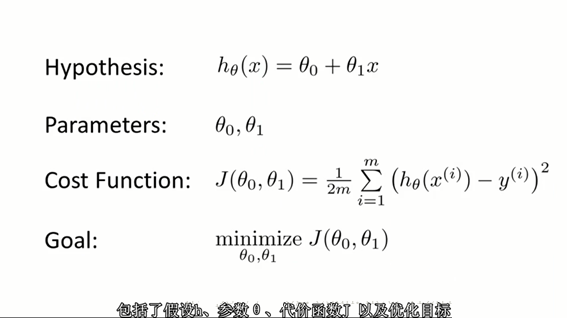3. 梯度下降：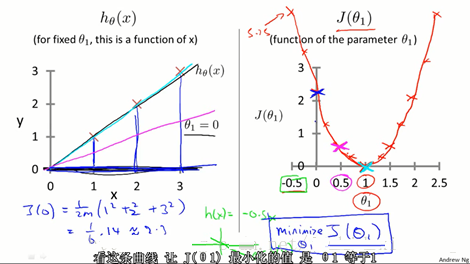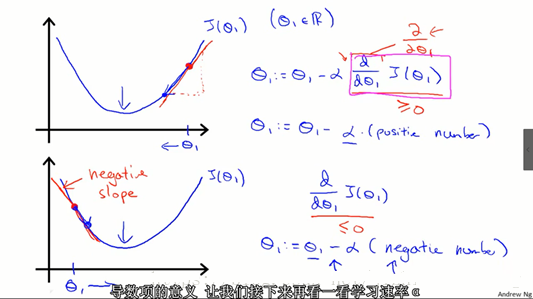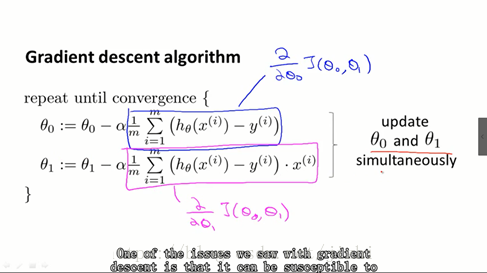4. Matlab实现：

 clc;clear;data = load('D:/Code/Data/ex1data1.txt');X = data(:,1);y = data(:,2);figure,plot(X, y, 'r*', 'MarkerSize', 5); % 5控制*的大小 % x加了一列,变成 (97,2)m = length(y); X = [ones(m, 1), data(:,1)]; % 初始化参数theta = zeros(2, 1); % Some gradient descent settingsiterations = 1500;alpha = 0.01;% 梯度下降，找到最佳参数theta = gradientDescent(X, y, theta, alpha, iterations); hold on; % keep previous plot visibleplot(X(:,2), X*theta, '-')legend('Training data', 'Linear regression')hold off

 function theta = gradientDescent(X, y, theta, alpha, num_iters) m = length(y); % 样本数量 for iter = 1:num_iters H = X * theta; %(97,2)*(2*1)=(97,1) Sum = [0 ; 0]; %(2,1)，记录偏导，求和 % theta_0更新 for i = 1 : m Sum(1,1) = Sum(1,1) + (H(i) - y(i)); end % theta_1更新 for i = 1 : m Sum(2,1) = Sum(2,1) + (H(i) - y(i)) * X(i,2)'; end theta = theta - (alpha * Sum) / m; end end

 Sum = [0 ; 0]; %(2,1)，记录偏导，求和 % theta_0更新 for i = 1 : m Sum(1,1) = Sum(1,1) + (H(i) - y(i)); end % theta_1更新 for i = 1 : m Sum(2,1) = Sum(2,1) + (H(i) - y(i)) * X(i,2)'; end theta = theta - (alpha * Sum) / m;

5. 效果如下：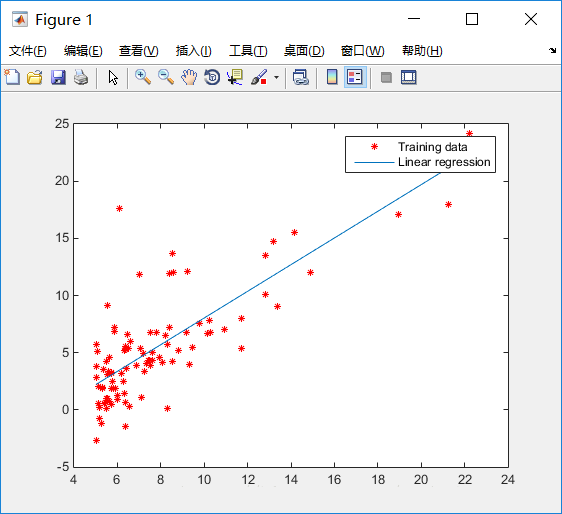1. 多变量的例子，如下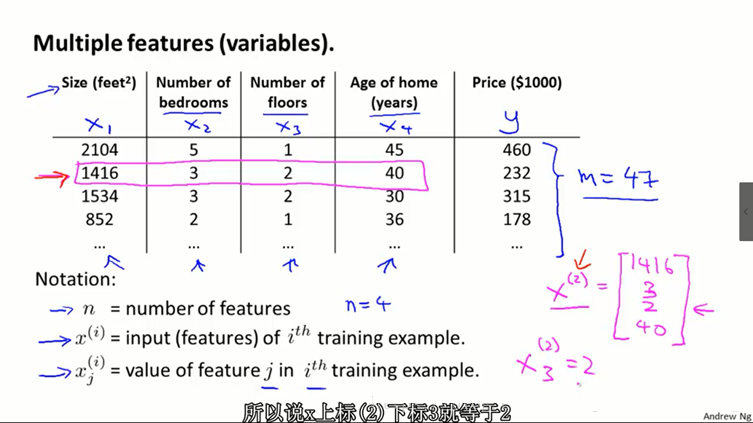2. 数据集下载点击打开链接，密码：ibtb：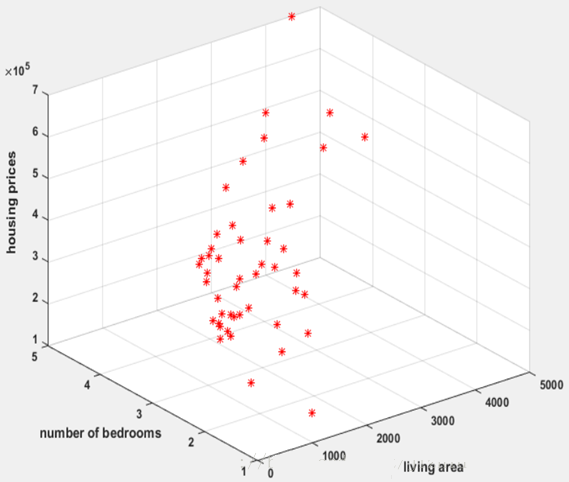3. 多变量的假设函数定义：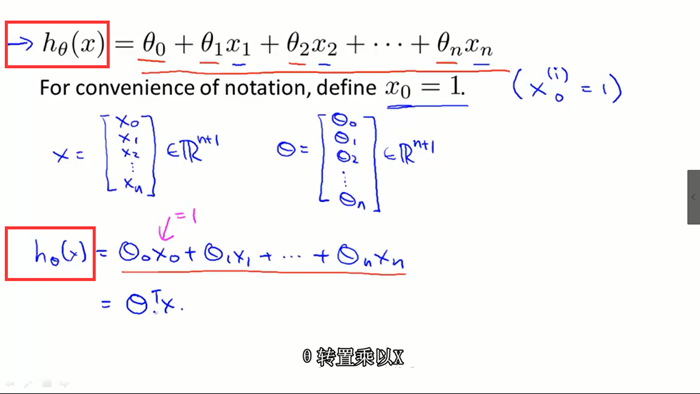4. 多变量的损失函数：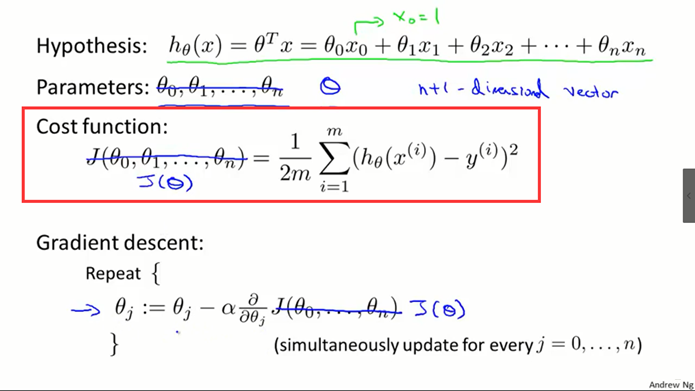5. 迭代过程如下：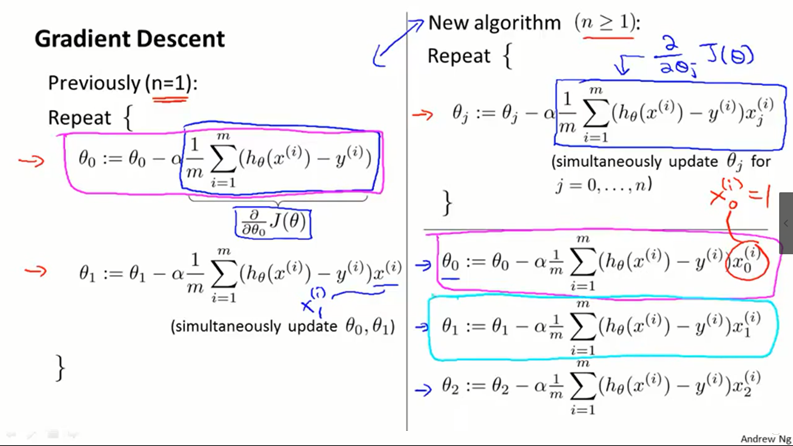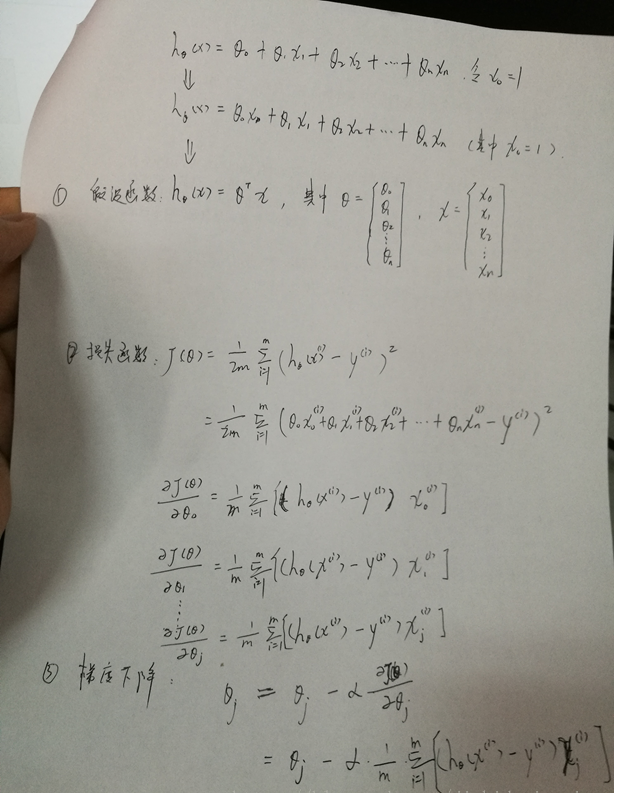6. 多变量的线性回归，Matlab实现：

 clear;clc; % 1，读取数据x = load('D:\Code\Data\ex3Data\ex3x.dat'); % 两个变量房子面积，和卧室数目，一行一个样本y = load('D:\Code\Data\ex3Data\ex3y.dat'); % 房子价格% plot3(x(:,1), x(:,2), y, 'r*');% grid on % 画上网格% hold on; % 后面将画上拟合的线 % 2, 处理数据m = length(x(:,1)); % 样本数x = [ones(m,1), x]; % x0 为1x(:,2) = (x(:,2) - mean(x(:,2))) ./ std(x(:,2));x(:,3) = (x(:,3) - mean(x(:,3))) ./ std(x(:,3));plot3(x(:,1), x(:,2), y, 'r*');grid on % 画上网格hold off; % 3，参数设置iter = 1500; % 迭代次数theta = zeros(1,3); % 线性函数的参数，为行向量alpha = 0.01; J = zeros(m,1);for i = 1:iter % 以迭代次数为终止条件 % 求以当前theta为线性函数的参数时，其损失值 h = x * theta'; % 假设函数 J(i) = 1/2*m * sum((h - y).^2); % 记录每次迭代后损失值情况，但对迭代终止没有影响 theta(1,1) = theta(1,1) - alpha*(1/m)* sum((h - y) .* x(:,1)); % x(:,1)即为x_0 theta(1,2) = theta(1,2) - alpha*(1/m)* sum((h - y) .* x(:,2)); theta(1,2) = theta(1,3) - alpha*(1/m)* sum((h - y) .* x(:,3)); % theta = theta - alpha*(1/m)* sum(x'*(h - y)); % 一次性处理所有theta end figure, % 画出损失函数随迭代次数的变化情况plot(1:iter, J);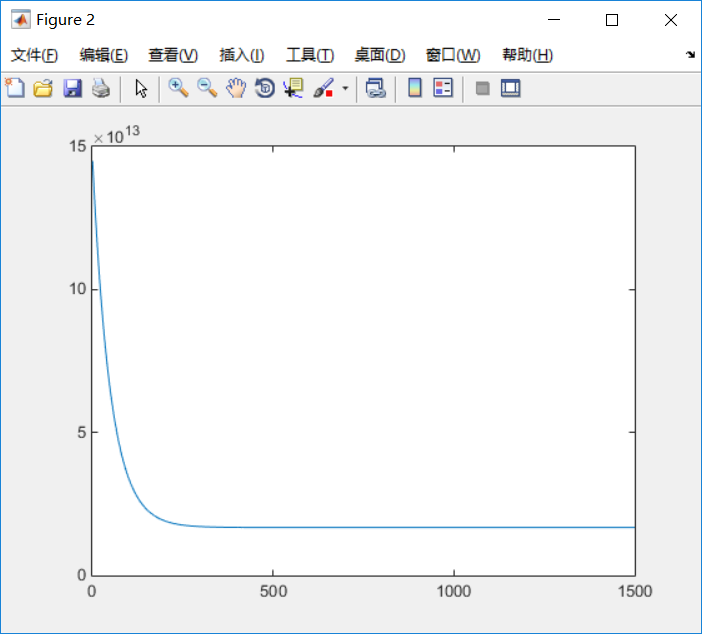theta(1,1) = theta(1,1) - alpha*(1/m)* sum((h - y) .* x(:,1)); % x(:,1)即为x_0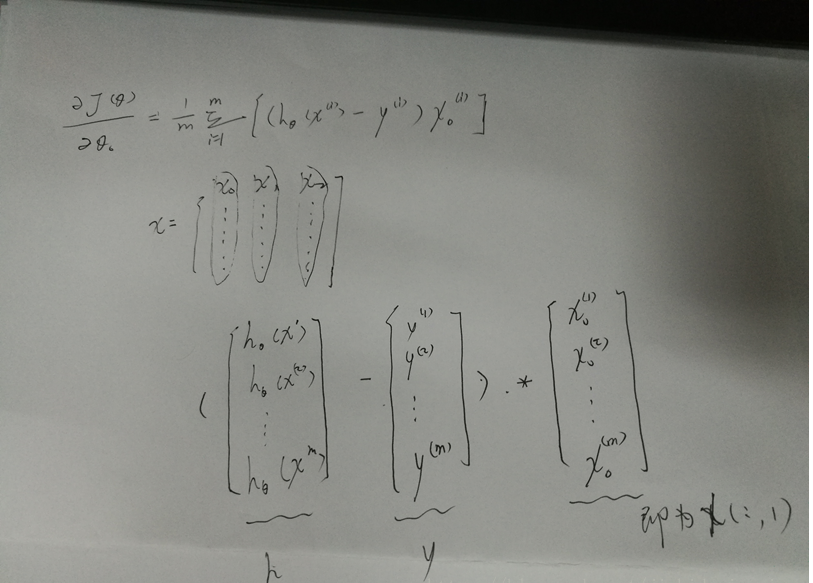1. 求最佳的参数theta，除了使用梯度下降法，也可以使用正规方程，具体方式，所有样本组成一个矩阵X，矩阵的每一行为一个样本， 参数theta直接使用下面的正规公式即可得到：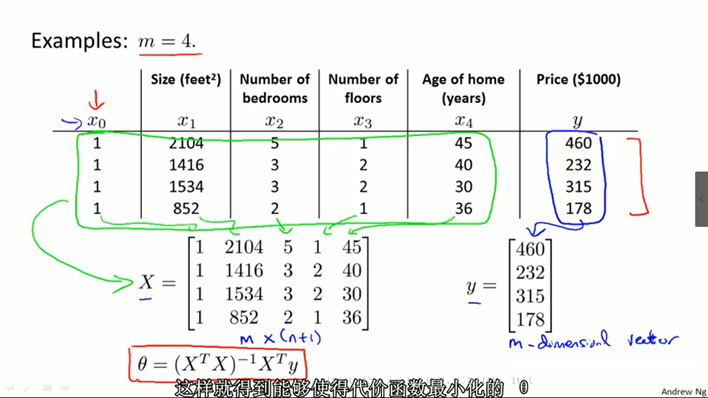2. 梯度下降法和正规方程对比：NG推荐特征数小于一万时，采用正规方程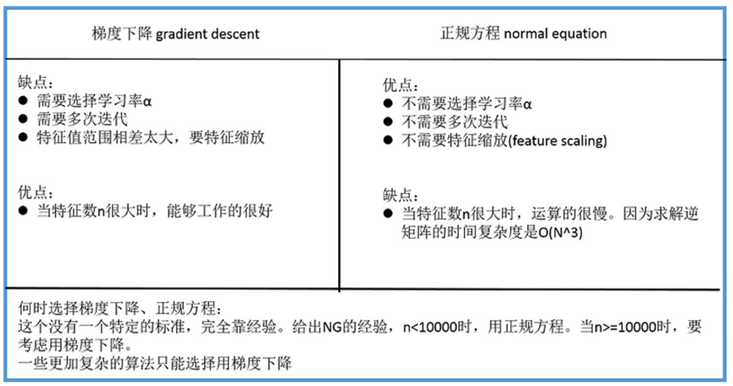3. 正规方程的推导过程：如果 y = X * theta，则损失函数值为0，即找到最佳theta。即已知y = X * theta，求theta？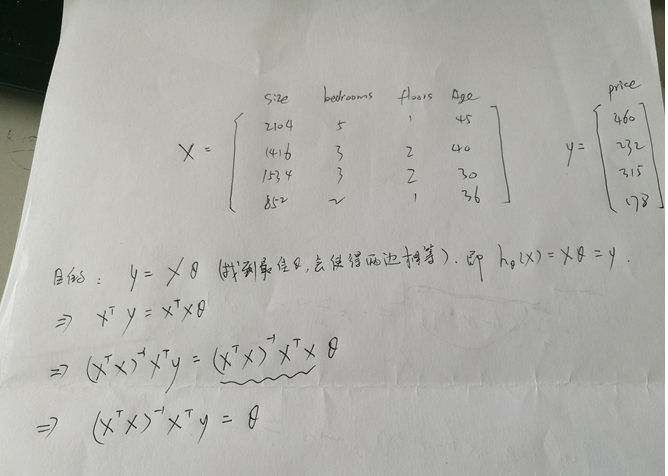1377 次浏览  评价: 好 中 差订阅捐助
 相关文章 我们该如何设计数据库 数据库设计经验谈 数据库设计过程 数据库编程总结
 相关文档 数据库性能调优技巧 数据库性能调整 数据库性能优化讲座 数据库系统性能调优系列
 相关课程 高性能数据库设计与优化 高级数据库架构师 数据仓库和数据挖掘技术 Hadoop原理、部署与性能调优每天2个文档/视频 扫描微信二维码订阅
 订阅技术月刊 获得每月300个技术资源